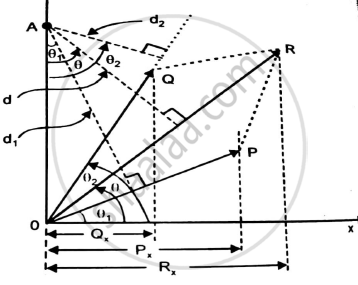# State and Prove Varigon’S Theorem. - Engineering Mechanics

state and prove varigon’s theorem.

#### Solution

ΣMAF= ΣMAR

Proof:Let P and Q be two concurrent forces at O,making angle θ1 and θ2 with the X-axis
Let R be the resultant making an angle θ with X axis
Let A be a point on the Y-axis about which we shall find the moments of P and Q and also of resultant R.
Let d1,d2 and d be the moment arm of P,Q and R from moment centre A
The x component of forces P,Q and R are Px,Qx and Rx
∴ MAP = P x d1 ……..(1)
∴ MAQ = Q x d2 ……..(2)
∴ MAR = R x d
=R( OA.cosθ )
=OA.Rx
∴ MAP+MAQ=Pd1+Qd2
ΣMAF = P x OAcosθ1 + Q x OAcos θ2
=OA.Px+OA.Qx (as Px=P.cosθ1 and Qx=Qcosθ2)
=OA(Px+Qx)
∴ ΣMAF = OA(Rx) ……..(3)
From (4) and (3)
ΣMAF= ΣMA
Thus,Varigon’s theorem is proved

Concept: Varignon’s Theorem
Is there an error in this question or solution?